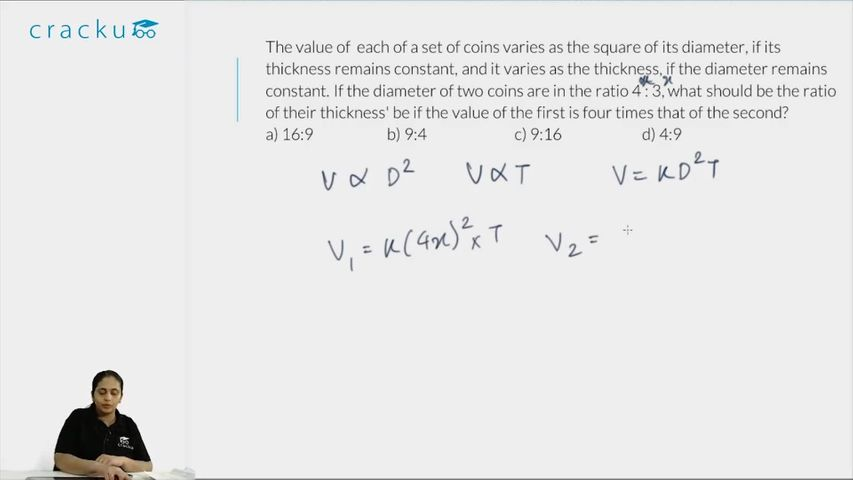Question 64

# The value of each of a set of coins varies as the square of its diameter, if its thickness remains constant, and it varies as the thickness, if the diameter remains constant. If the diameter of two coins are in the ratio 4 : 3, what should be the ratio of their thickness' be if the value of the first is four times that of the second?

Solution

Value of coin = $$k (2r)^2 t$$ (where k is proportionality constant, 2r is diameter and t is thickness)
So (value of first coin) = 4 (value of second coin)

$$k (2r_1)^2 t_1 = 4 \times (k(2r_2)^2 t_2)$$

or $$\frac{t_1}{t_2} = \frac{9}{4}$$  (As ratio of diameters 2r will be 9:4)

### View Video Solution